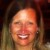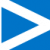# Attn Minitab 17 Experts: Calculating Confidence Intervals in Graphical Summaries

Six Sigma – iSixSigma Forums General Forums Tools & Templates Attn Minitab 17 Experts: Calculating Confidence Intervals in Graphical Summaries

Viewing 4 posts - 1 through 4 (of 4 total)
• Author
Posts
• #236393tgause
Participant

As I was playing around in Minitab 17 today, I noticed that my hand-calculated confidence interval (CI) for a dataset did not match the CI that Minitab calculated for the same dataset. (Please don’t ask why I’m hand calculating this stuff. Glutton for punishment, I guess.) In any case, my dataset has over 30 records, therefore, I was using the z-table to calculate the CI. Rationale: Dataset sample is large (n>=30) and data is normal (Minitab normality test rejected the null hypothesis). Therefore, I can use z-distribution table. I got an Upper Limit of \$99.94 and a Lower Limit of \$69.00.

However, when I plugged this same data into Minitab and ran a Graphical Summary (Stat > Basic Statistics > Graphical Summary), it gave me the following: UL=\$101.06 and LL=\$67.87. Weird, right? So, I recalculated the CI again, by hand (remember, glutton for punishment), but this time I used the Student t-distribution and realized that, even though the sample is greater than 30, Minitab used the Student t-distribution to calculate the CI.

Now that I know this, I’ll be aware of it. Can anyone explain why, though? I’m just curious, that’s all.

0
#236395Katie Barry
Keymaster

@minitab Can you weigh in?

0
#236406Minitab
Participant

Hi Tom. I’ll be glad to clarify. The sample mean has an exact standard normal (Z) distribution when the population from which the sample is selected is normally distributed with known standard deviation. That’s a helpful academic consideration when learning about confidence intervals, but, in practice, we almost never know the true standard deviation.

In the vast majority of cases, we have to estimate the standard deviation from the sample data, just as we do the mean. In those cases, even when the sample size is “large” (n > 30-40 in most introductory statistics books), the sample mean is approximately normally distributed. That approximation is helpful in academic settings that require manual calculations, e.g. it is much easier to look up a z-multiplier than a t-multiplier when using tables. However, with access to Minitab and incredible computing power, settling for an approximation is unnecessary. We utilize the t-distribution to create a more exact confidence interval.

Further, the confidence interval for the mean based on the t-distribution typically works well even when the population isn’t normally distributed, unless the population distribution is highly skewed and the sample size (n) is very small.

If you would like, you can still calculate a confidence interval for a mean based on the standard normal (Z) distribution via Stat > Basic Statistics > 1-Sample Z. You will notice, however, that the dialog requires you to specify the known standard deviation of the population.

Finally, you mentioned that you believe that your specific data are normally distributed because Minitab rejected the null of the normality test. The null hypothesis of a normality test is that the normal distribution fits the data. Thus, if the p-value from the test is small and the null is rejected, the conclusion is actually that the normal distribution does not fit the data.

1
#236700tgause
Participant

@Minitab – First, thank you for taking the time to respond to my question and for clearly explaining what was going on. Second, based on your response, I realized I had made an error in my post. I should have said the normality test “failed to reject” the null hypothesis.

0
Viewing 4 posts - 1 through 4 (of 4 total)

You must be logged in to reply to this topic.